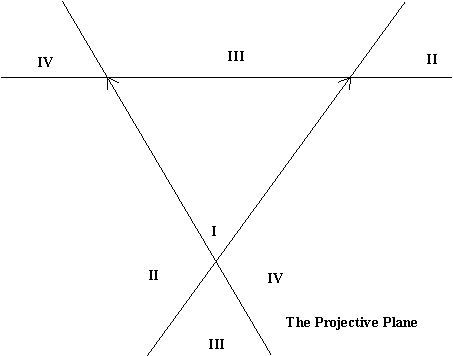Math 105
Thursday,  April 27

Review from last class:
What is the calculus?

A systematic method for answering these questions of velocity, tangents, change in position and area that uses the algebraic representation of a relation between y and x .
Examples: if y = .... then the slope of the tangent to the curve described by this relationship at the point (a,b) is....
If the position s = ... t.... then the instantaneous velocity of the object moving to correspond to this relation  at time t is .................
If the velocity of a moving object is given by v= .... t..... , then the object has a net change during the interval from t = a to t = b of .....
the area of the region in the plane bounded by the Xis, the lines X = a and X= b and the graph of y= ...x... is....

Newton: Tangent lines, velocity, and the derivative.
Using systematic  estimates to find i) the slope of the tangent line and ii) the instantaneous velocity.
The derivative: a limiting number interpreted as
i) the slope of the tangent line or
ii) the instantaneous velocity
A calculus for derivatives: core functions- example powers of x.
Rules: sum . constant multiples, etc. [Ways that we put together core functions]
Activity: Finding the derivative of a function by estimation and by rules

Application: Finding maximum - old Macdonald: Fence Problem- find dimension of largest rectangular area enclosed by a fence of length 100 meters using the side of a barn as part of the boundary of the rectangle.
A = f (x).
What does the maximium area have to do with the slope of the tangents to the graph of A?
When is tangent horizontal? calculus - formula for slope of the tangent as a function of x: ...

Notation: y = f(x). dy/dx = f'(x) Leibniz.Lagrange

Reversing  the process- given the derivative- find the original function(s): "Integration".
Interpretation: Given slopes of tangent lines- fit a curve to them. [A dot to dot problem.]
Given velocity, find a position motion that corresponds. [A detective problem]
Determining position and areas. Change in position - area of rectangle.
Putting concepts together with computations.
To find area under curve y = v(x). Find s where s' = v. Area corresponds to net change in position.
Fundamental Theorem of Calculus: Area = s(b) - s(a).
Notation: v = F '(x); int v dx = F(x) + C; int_a^b v dx = F(b) - F(a)

"Seeing the Infinite"

Seeing the infinite... large.

How to see the Infinite using "Projective geometry".

We will try to understand
• why we see the horizon on a plane....
• why the horizon is a line composed of ideal/infinite points.
• why parallel lines "meet" on the horizon.

Center of projection/ perspective.
[compare with similarity and geometric series].
Correspondences:

Two Line segments of different length.

Semicircle to line and line to semicircle!
Two points at "infinity"
Circle to line and line to Circle!
One point at "infinity"

How to see the Infinite on a line using Projective geometry.
Line to Line

Use Rope and lines on floor with an image plane to see parallels meet on an "image" plane ---
where is the horizon?

Perpective between planes in Space!

Seeing the horizon on a plane.... a line of ideal/infinite points.

How parallel lines "meet" on the horizon.
What is the Affine Plane? the Projective Plane?

How do we see curves in perspective, drawn in the Projective Plane?
Using coordinates to draw in perspective.

Creating coordinates in the affine plane.
Question: What is on the other side of the horizon?

Distinguish: The affine plane from the projective plane.

Using coordinates to transfer a circle to the affine plane as an ellipse.Equations for conics in coordinate geometry.Using coordinates to draw in perspective.

What do the conics look like in a perspective drawing or in the projective plane?Some final remarks on the projective plane and visualizing Flatland and Space.:

• What is on the other side of the horizon?
• The projective plane as a surface.
• Visualizing the projective plane on a disc with the boundary identified as the horizon.
• The projective plane as a non-euclidean flatland.